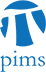## Discrete Math Seminar: Computing solution concepts in games with integer decisions

• Date: 03/16/2010
Lecturer(s):
Chris Ryan (UBC)
Location:

University of Alberta

Description:

I discuss algorithms and complexity results for two game theoretic extensions of integer programming: integer programming games and bilevel integer programming. In the case of integer programming games, I discuss an algorithm which computes pure Nash equilibria using rational generating functions which runs in polynomial time when certain parameters are fixed. In the case of bilevel integer programming, I describe an algorithm which decides the existence of and computes optimistic" optimal solutions using parametric integer programming and binary search. I show that this algorithm runs in polynomial time when the number of integer variables are fixed, extending a result by Lenstra on integer programming in fixed dimension to the bilevel setting.

This is joint work with Matthias Koeppe and Maurice Queyranne.

Schedule:

4:00 - 5:00pm, WMAX 216.